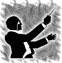# m4v

Members

847

## Community Reputation

627 Excellent

• Rank
Junior Rocket Scientist

## Recent Profile Visitors

The recent visitors block is disabled and is not being shown to other users.

1. I applied the definition of potential field, which is the amount of work needed to move a mass m from a zero potential (this case the center of Earth) to the point of interest, that the result is equal to kinetic energy makes total sense, since the centrifugal force is a fictitious force that arises due to the angular speed of an object. Now I recognize you're using the potential energy of the near earth gravity equation but instead of the gravity acceleration you're putting centrifugal acceleration. Yeah, I wouldn't mix them like that since they aren't analogous, for one the gravitational force decreases as you increase R while the centrifugal increases.
2. But you have R_pole in there, it shouldn't be there if there's just the centrifugal term at the equator (centripetal points to the center, centrifugal outwards) so what it is? how did you get it? what values are you using? I did the integral myself, and I get 0.5*m(w R)^2, (w being angular velocity in radians, V=wR), calculating that for the equator you get 108kJ/kg so half of the 200kJ/kg you said before. Since is in the order of magnitude I would say that's good enough, we aren't going to get exact numbers with the approximations we're using. We would need to know the math for calculating the shape of bodies at hydrostatic equilibrium for get a complete answer.
3. no, I don't know what this term "mV^2/R_equator*(R_equator - R_pole)" is supposed to mean, is the integral of the centrifugal force for put it in units of energy? And the centrifugal force depends of the distance from the rotating axis, not the center of Earth, at the poles it would be zero.
• 61 replies
• 1
•4. When you add centrifugal forces it deforms that potential field into a spheroid. You cannot consider gravitational potential alone when in a rotating frame such as Earth, this is why we usually talk about gravity and not gravitation.
5. Sea level is at constant potential energy, nobody said that that has to be spherical, it is a spheroid. At the poles the centrifugal forces are lower thus the gravity is stronger, at equator the centrifugal force is maximum thus the gravity is weaker and the potential level you have at the poles ends up higher.
6. Sea level is literally the sea level, it isn't the radius of a imagined sphere, it's also oblate. The rotation of the planet produces centrifugal forces that cancels a bit of the gravitational potential, this force is strongest at the equator so stuff weight less there, thus the planet's bulge.
• 61 replies
• 1
•7.## [1.5.x] Engine Tweaks for Making History [Deprecated]

More than a vacuum engine, the Poddle is an engine for landers, that's why the bell is so small (same reason with the terrier), so you can have some clearance while landing. It shouldn't be hidden by default, you are limiting options for landers.
8. I'm real busy, so don't expect an update soon.
• 926 replies
• 3
•9. @StahnAileronFeature noted, i don't think it would be hard to do, but development is in hiatus for the moment and I have some backlog in my TODO list.
10. @ErrolI have a feature like that in mind but I'm not actively working in RCSBA these days. torque magnitude.
11. if you can put a screenshot we might have a better idea of what is going on. edit: maybe the window is minimized, click the small button in the right top corner of the window, is a little square.
12. The red circular arrow represents the angular acceleration, the straight red arrow is the torque vector. Torque is always represented as a vector that points along the rotation axis. In practice you don't need to give much attention to that vector though.
13. "drycom_<resource>" is just a variable for remembering if a given resource should/shouldn't accounted for the DCoM calculation. If a mod adds resources then RCSBA saves a setting for it.
14. yes it does, for some reason...
15. Version 0.9.1 ------------- * fix plugin button. also added Toolbar's support back.